# 7.1 Greatest common factor and factor by grouping  (Page 2/5)

 Page 2 / 5
$\begin{array}{cccc}\hfill 2\left(x+7\right)\hfill & & & \text{factors}\hfill \\ \hfill 2·x+2·7\hfill & & & \\ \hfill 2x+14\hfill & & & \text{product}\hfill \end{array}$

Now we will start with a product, like $2x+14$ , and end with its factors, $2\left(x+7\right)$ . To do this we apply the Distributive Property “in reverse.”

We state the Distributive Property here just as you saw it in earlier chapters and “in reverse.”

## Distributive property

If $a,b,c$ are real numbers, then

$\begin{array}{ccccccc}a\left(b+c\right)=ab+ac\hfill & & & \text{and}\hfill & & & ab+ac=a\left(b+c\right)\hfill \end{array}$

The form on the left is used to multiply. The form on the right is used to factor.

So how do you use the Distributive Property to factor a polynomial? You just find the GCF of all the terms and write the polynomial as a product!

## How to factor the greatest common factor from a polynomial

Factor: $4x+12$ .

## Solution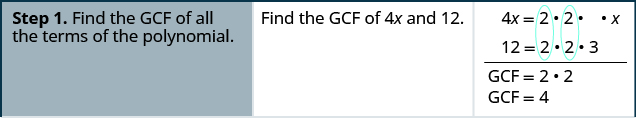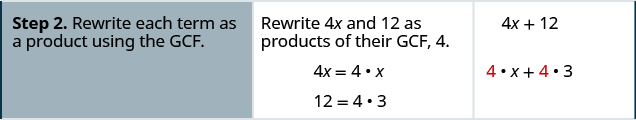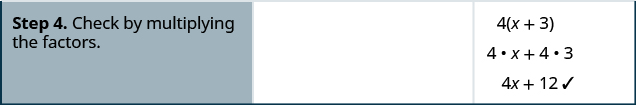Factor: $6a+24$ .

$6\left(a+4\right)$

Factor: $2b+14$ .

$2\left(b+7\right)$

## Factor the greatest common factor from a polynomial.

1. Find the GCF of all the terms of the polynomial.
2. Rewrite each term as a product using the GCF.
3. Use the “reverse” Distributive Property to factor the expression.
4. Check by multiplying the factors.

## Factor as a noun and a verb

We use “factor” as both a noun and a verb.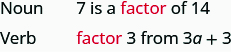Factor: $5a+5$ .

## Solution

 Find the GCF of 5 a and 5.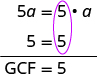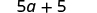Rewrite each term as a product using the GCF.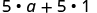Use the Distributive Property "in reverse" to factor the GCF.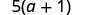Check by mulitplying the factors to get the orginal polynomial. $5\left(a+1\right)$ $5\cdot a+5\cdot 1$ $5a+5✓$

Factor: $14x+14$ .

$14\left(x+1\right)$

Factor: $12p+12$ .

$12\left(p+1\right)$

The expressions in the next example have several factors in common. Remember to write the GCF as the product of all the common factors.

Factor: $12x-60$ .

## Solution

 Find the GCF of 12 x and 60.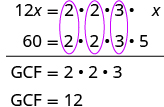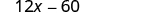Rewrite each term as a product using the GCF.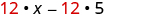Factor the GCF.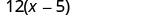Check by mulitplying the factors. $12\left(x-5\right)$ $12\cdot x-12\cdot 5$ $12x-60✓$

Factor: $18u-36$ .

$8\left(u-2\right)$

Factor: $30y-60$ .

$30\left(y-2\right)$

Now we’ll factor the greatest common factor from a trinomial. We start by finding the GCF of all three terms.

Factor: $4{y}^{2}+24y+28$ .

## Solution

We start by finding the GCF of all three terms.

 Find the GCF of $4{y}^{2}$ , $24y$ and 28.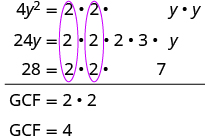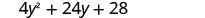Rewrite each term as a product using the GCF.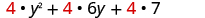Factor the GCF.Check by mulitplying. $4\left({y}^{2}+6y+7\right)$ $4\cdot {y}^{2}+4\cdot 6y+4\cdot 7$ $4{y}^{2}+24y+28✓$

Factor: $5{x}^{2}-25x+15$ .

$5\left({x}^{2}-5x+3\right)$

Factor: $3{y}^{2}-12y+27$ .

$3\left({y}^{2}-4y+9\right)$

Factor: $5{x}^{3}-25{x}^{2}$ .

## Solution

 Find the GCF of $5{x}^{3}$ and $25{x}^{2}.$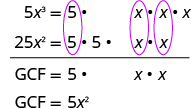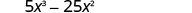Rewrite each term.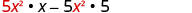Factor the GCF.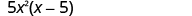Check. $5{x}^{2}\left(x-5\right)$ $5{x}^{2}\cdot x-5{x}^{2}\cdot 5$ $5{x}^{3}-25{x}^{2}✓$

Factor: $2{x}^{3}+12{x}^{2}$ .

$2{x}^{2}\left(x+6\right)$

Factor: $6{y}^{3}-15{y}^{2}$ .

$3{y}^{2}\left(2y-5\right)$

Factor: $21{x}^{3}-9{x}^{2}+15x$ .

## Solution

In a previous example we found the GCF of $21{x}^{3},9{x}^{2},15x$ to be $3x$ .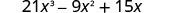Rewrite each term using the GCF, 3 x .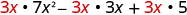Factor the GCF.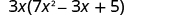Check. $3x\left(7{x}^{2}-3x+5\right)$ $3x\cdot 7{x}^{2}-3x\cdot 3x+3x\cdot 5$ $21{x}^{3}-9{x}^{2}+15x✓$

Factor: $20{x}^{3}-10{x}^{2}+14x$ .

$2x\left(10{x}^{2}-5x+7\right)$

Factor: $24{y}^{3}-12{y}^{2}-20y$ .

$4y\left(6{y}^{2}-3y-5\right)$

Factor: $8{m}^{3}-12{m}^{2}n+20m{n}^{2}$ .

## Solution

 Find the GCF of $8{m}^{3}$ , $12{m}^{2}n$ , $20m{n}^{2}$ .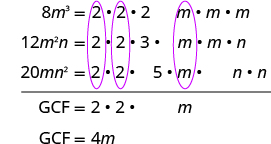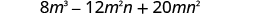Rewrite each term.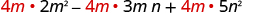Factor the GCF.Check. $4m\left(2{m}^{2}-3mn+5{n}^{2}\right)$ $4m\cdot 2{m}^{2}-4m\cdot 3mn+4m\cdot 5{n}^{2}$ $8{m}^{3}-12{m}^{2}n+20m{n}^{2}✓$

Factor: $9x{y}^{2}+6{x}^{2}{y}^{2}+21{y}^{3}$ .

$3{y}^{2}\left(3x+2{x}^{2}+7y\right)$

Factor: $3{p}^{3}-6{p}^{2}q+9p{q}^{3}$ .

$3p\left({p}^{2}-2pq+3{q}^{2}\right)$

When the leading coefficient is negative, we factor the negative out as part of the GCF.

Factor: $-8y-24$ .

## Solution

When the leading coefficient is negative, the GCF will be negative.

 Ignoring the signs of the terms, we first find the GCF of 8 y and 24 is 8. Since the expression −8 y − 24 has a negative leading coefficient, we use −8 as the GCF.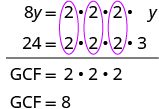Rewrite each term using the GCF.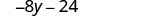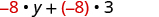Factor the GCF.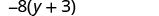Check. $-8\left(y+3\right)$ $-8\cdot y+\left(-8\right)\cdot 3$ $-8y-24✓$

Aziza is solving this equation-2(1+x)=4x+10
No. 3^32 -1 has exactly two divisors greater than 75 and less than 85 what is their product?
x^2+7x-19=0 has Two solutions A and B give your answer to 3 decimal places
3. When Jenna spent 10 minutes on the elliptical trainer and then did circuit training for20 minutes, her fitness app says she burned 278 calories. When she spent 20 minutes onthe elliptical trainer and 30 minutes circuit training she burned 473 calories. How manycalories does she burn for each minute on the elliptical trainer? How many calories doesshe burn for each minute of circuit training?
.473
Angelita
?
Angelita
John left his house in Irvine at 8:35 am to drive to a meeting in Los Angeles, 45 miles away. He arrived at the meeting at 9:50. At 3:30 pm, he left the meeting and drove home. He arrived home at 5:18.
p-2/3=5/6 how do I solve it with explanation pls
P=3/2
Vanarith
1/2p2-2/3p=5p/6
James
Cindy
4.5
Ruth
is y=7/5 a solution of 5y+3=10y-4
yes
James
Cindy
Lucinda has a pocketful of dimes and quarters with a value of $6.20. The number of dimes is 18 more than 3 times the number of quarters. How many dimes and how many quarters does Lucinda have? Rhonda Reply Find an equation for the line that passes through the point P ( 0 , − 4 ) and has a slope 8/9 . Gabriel Reply is that a negative 4 or positive 4? Felix y = mx + b Felix if negative -4, then -4=8/9(0) + b Felix -4=b Felix if positive 4, then 4=b Felix then plug in y=8/9x - 4 or y=8/9x+4 Felix Macario is making 12 pounds of nut mixture with macadamia nuts and almonds. macadamia nuts cost$9 per pound and almonds cost $5.25 per pound. how many pounds of macadamia nuts and how many pounds of almonds should macario use for the mixture to cost$6.50 per pound to make?
Nga and Lauren bought a chest at a flea market for $50. They re-finished it and then added a 350 % mark - up Makaila Reply$1750
Cindy
the sum of two Numbers is 19 and their difference is 15
2, 17
Jose
interesting
saw
4,2
Cindy
Felecia left her home to visit her daughter, driving 45mph. Her husband waited for the dog sitter to arrive and left home 20 minutes, or 13 hour later. He drove 55mph to catch up to Felecia. How long before he reaches her?
hola saben como aser un valor de la expresión
NAILEA
integer greater than 2 and less than 12
2 < x < 12
Felix
I'm guessing you are doing inequalities...
Felix
Actually, translating words into algebraic expressions / equations...
Felix
hi
Darianna
hello
Mister
Eric here
Eric
6
Cindy

#### Get Jobilize Job Search Mobile App in your pocket Now!By Sandhills MLTBy Sandhills MLTBy John GabrieliBy Dan ArielyBy Anh DaoBy David CoreyBy Steve GibbsBy Lakeima RobertsBy Richley CrapoBy OpenStax